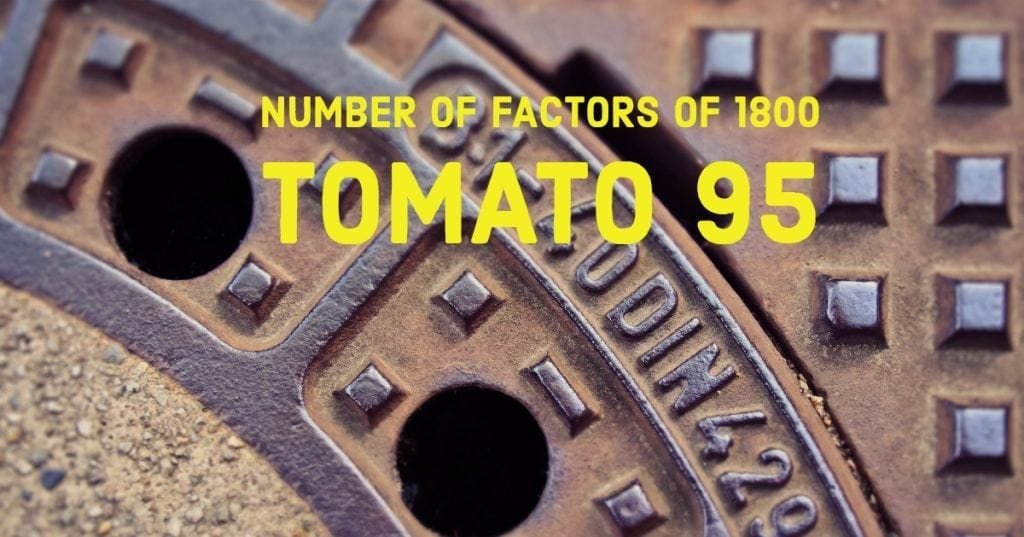How Cheenta works to ensure student success?
Explore the Back-Story

# Number of factors of 1800 | Tomato Problem 95This is a problem number 95 from TOMATO based on finding the Number of factors of 1800.

Problem
The number of different factors of $1800$ equals:
(A) $12$; (B) $210$; (C) $36$; (D) $18$;

Discussion:
We may factor $1800$ as $2^3 \times 3^2 \times 5^2$
Then the number of factors is: $(3+1) \times (2+1) \times (2+1) = 36$

Hence answer is $36$.

## Chatuspathi:

• What is this topic: Number Theory
• What are some of the associated concept: Number Theoretic Functions
• Where can learn these topics: Cheenta I.S.I. & C.M.I. course, discusses these topics in the ‘Number Theory’ module.
• Book Suggestions: Elementary Number Theory by David Burton

This is a problem number 95 from TOMATO based on finding the Number of factors of 1800.

Problem
The number of different factors of $1800$ equals:
(A) $12$; (B) $210$; (C) $36$; (D) $18$;

Discussion:
We may factor $1800$ as $2^3 \times 3^2 \times 5^2$
Then the number of factors is: $(3+1) \times (2+1) \times (2+1) = 36$

Hence answer is $36$.

## Chatuspathi:

• What is this topic: Number Theory
• What are some of the associated concept: Number Theoretic Functions
• Where can learn these topics: Cheenta I.S.I. & C.M.I. course, discusses these topics in the ‘Number Theory’ module.
• Book Suggestions: Elementary Number Theory by David Burton

This site uses Akismet to reduce spam. Learn how your comment data is processed.

### Knowledge Partner